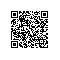# 堆排序

/**
* 堆排序类
*
* @author ln
* @email lining90567@sina.com
*/
public class HeapSort {

private int[] numbers;

/**
* 构造函数
*
* @param numbers
*            待排序数组
*/
public HeapSort(int[] numbers) {
this.numbers = numbers;
}

public void sort() {
buildMaxHeapify(this.numbers);
heapSort(this.numbers);
}

/**
* 构建最大堆
*
* @param numbers
*            待排序数组
*/
private void buildMaxHeapify(int[] numbers) {
// 没有子节点的才需要创建最大堆，从最后一个的父节点开始
int startIndex = getParentIndex(numbers.length - 1);
// 从尾端开始创建最大堆，每次都是正确的堆
for (int i = startIndex; i >= 0; i--) {
maxHeapify(numbers, numbers.length, i);
}
}

/**
* 创建最大堆
*
* @param numbers
*            待排序数组
* @param heapSize
*            需要创建最大堆的大小，一般在sort的时候用到，因为最多值放在末尾，末尾就不再归入最大堆了
* @param index
*            当前需要创建最大堆的位置
*/
private void maxHeapify(int[] numbers, int heapSize, int index) {
// 当前点与左右子节点比较
int left = getChildLeftIndex(index);
int right = getChildRightIndex(index);

int largest = index;
if (left < heapSize && numbers[index] < numbers[left]) {
largest = left;
}
if (right < heapSize && numbers[largest] < numbers[right]) {
largest = right;
}
// 得到最大值后可能需要交换，如果交换了，其子节点可能就不是最大堆了，需要重新调整
if (largest != index) {
int temp = numbers[index];
numbers[index] = numbers[largest];
numbers[largest] = temp;
maxHeapify(numbers, heapSize, largest);
}
}

/**
* 排序，最大值放在末尾，data虽然是最大堆，在排序后就成了递增的
*
* @param numbers
*            待排序数组
*/
private void heapSort(int[] numbers) {
// 末尾与头交换，交换后调整最大堆
for (int i = numbers.length - 1; i > 0; i--) {
int temp = numbers;
numbers = numbers[i];
numbers[i] = temp;
maxHeapify(numbers, i, 0);
}
}

/**
* 父节点位置
*
* @paramcurrent
* @return
*/
private int getParentIndex(int current) {
return (current - 1) >> 1;
}

/**
* 左子节点position注意括号，加法优先级更高
*
* @paramcurrent
* @return
*/
private int getChildLeftIndex(int current) {
return (current << 1) + 1;
}

/**
* 右子节点position
*
* @paramcurrent
* @return
*/
private int getChildRightIndex(int current) {
return (current << 1) + 2;
}

}使用钉钉扫一扫加入圈子
+ 订阅